Support the Monkey! Tell All your Friends and TeachersHome MonkeyNotes Printable Notes Digital Library Study Guides Study Smart Parents Tips College Planning Test Prep Fun Zone Help / FAQ How to Cite Request a New Title

8.11 Sampling Of Variables

The sample mean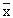is normally distributed with mean m and standard deviation s /Ön where m is the mean of the population and s is the standard deviation of the population. This results will help us in testing a hypothesis about the population mean.

1. Testing the hypothesis that the population mean m = m0 which is a specified value.

Now s = S.D. of the population is known.

In notations :-

The null hypothesis : m = m0 i.e. m - m0 = 0. First calculate1. If | z | < 1.96, the difference is not significant at 5% level and Ho is accepted, otherwise rejected.

2. If | z | < 2.58, the difference is not significant at 1% level and Ho is accepted, otherwise rejected.

Note: We have assumed that s is known. If however s is not known, we take s to be equal to the S.D. of the sample.

Example A random sample of 400 male students have average weight of 55 kg. Can we say that the sample comes from a population with mean 58 kg. with a variance of 9 kg. ?Your browser does not support the IFRAME tag.

Solution: The null hypothesis Ho is that the sample comes from the given population.

In notations : Ho : m = 58 kg. and Ha : m ¹ 58 kg.

Now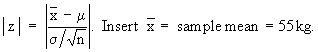m = population mean = 58 kg. n = 400 and s = population S.D. = 3

Therefore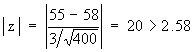This value is highly significant. We will reject Ho on the basis of this sample. The sample therefore, is not likely to be from the given population.

Example A random sample of 400 tins of vegetable oil and labeled "5 kg. net weight" has a mean net weight of 4.98 kg. with standard deviation of 0.22 kg. Do we reject the hypothesis of net weight of 5 kg. per tin on the basis of this sample at 1% level of significance ?

Solution: The null hypothesis Ho is that the net weight of each tin is 5 kg.

In notations Ho : m = 5 kg.

Inserting,= 4.98 kg., m = 5 kg. s = 0.22 kg. and n = 400

In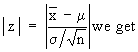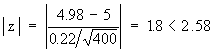Hence Ho is accepted at 1% level of significance.

Index

8.1 Population
8.2 Sample
8.3 Parameters and Statistic
8.4 Sampling Distribution
8.5 Sampling Error
8.6 Central Limit Theorem
8.7 Critical Region
8.8 Testing of Hypothesis
8.9 Errors in Tesitng of Hypothesis
8.10 Power o a Hypothesis Test
8.11 Sampling of Variables
8.12 Sampling of Attributes
8.13 Estimation
8.14 Testing the Difference Between Means
8.15 Test for Difference Between Proportions
8.16 Two Tailed and one Tailed Tests
8.17 Test of Significance for Small Samples
8.18 Students t-distribution
8.19 Distribution of 't' for Comparison of Two Samples Means Independent Samples
8.20 Testing Difference Between Mens of Two Samples Dependent Samples or Matched Paired Observations
8.21 Chi-Square
8.22 Sampling Theory of Correlation
8.23 Sampling Theory of Regression

Chapter 1Search: All Products Books Popular Music Classical Music Video DVD Toys & Games Electronics Software Tools & Hardware Outdoor Living Kitchen & Housewares Camera & Photo Cell Phones Keywords: